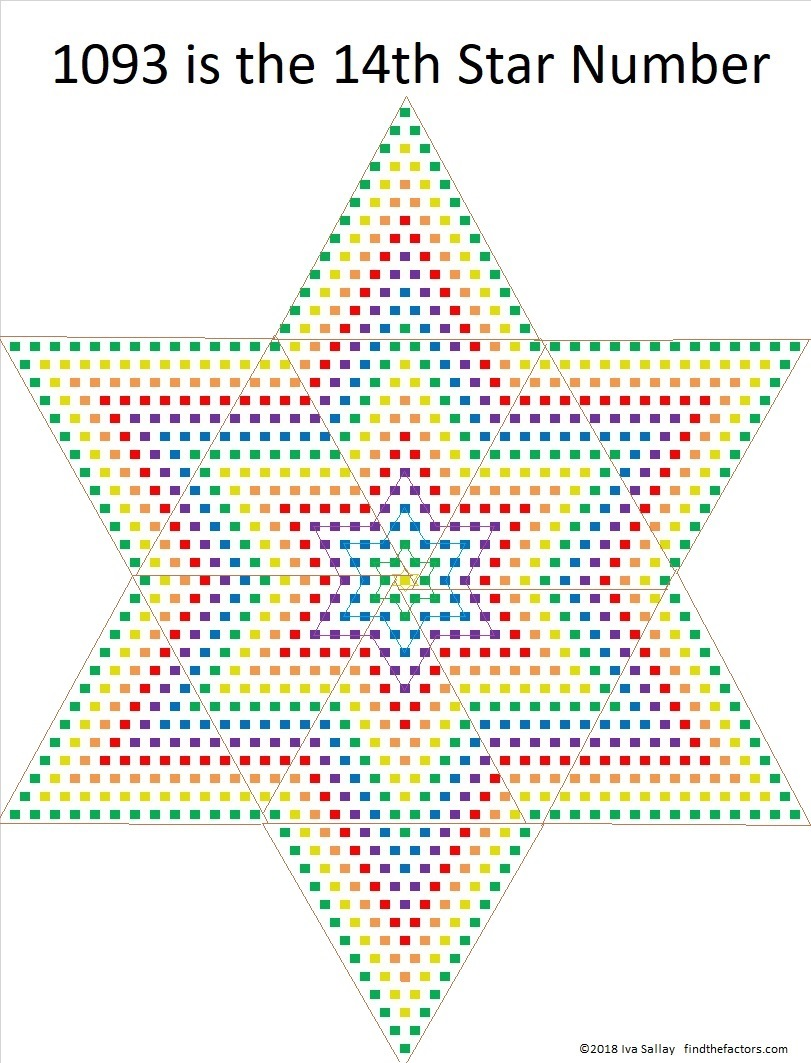# 1093 is a STAR!

(12 × 13 × 14)/2 = 1092 and that makes 1093 a STAR!1092 had more than its fair share of factors forcing 1093 to have only two factors, but that’s okay because 1093 is a beautiful STAR! Why is it a STAR? Because 1093 is one more than 12 times the 13th triangular number. Do you see those 12 triangles in the image above? Each of them has the same number of tiny squares. The yellow square in the center is the plus one that completes the star.

There are some other reasons why 1093 deserves a gold star:

Not every prime number is in a twin prime, but 1091 and 1093 are twin primes. Even fewer are part of a prime triplet, but those twin primes are part of TWO prime triplets: the 31st and the 32nd! That’s because 1087, 1091, 1093, and 1097 are all prime numbers.

• 1093 is a prime number.
• Prime factorization: 1093 is prime.
• The exponent of prime number 1093 is 1. Adding 1 to that exponent we get (1 + 1) = 2. Therefore 1093 has exactly 2 factors.
• Factors of 1093: 1, 1093
• Factor pairs: 1093 = 1 × 1093
• 1093 has no square factors that allow its square root to be simplified. √1093 ≈ 33.03329

How do we know that 1093 is a prime number? If 1093 were not a prime number, then it would be divisible by at least one prime number less than or equal to √1093 ≈ 33.1. Since 1093 cannot be divided evenly by 2, 3, 5, 7, 11, 13, 17, 19, 23, 29 or 31, we know that 1093 is a prime number.1093 is the sum of two squares:
33² + 2² = 1093

It is the hypotenuse of a primitive Pythagorean triple:
132-1085-1093 calculated from 2(33)(2), 33² – 2², 33² + 2²

Here’s another way we know that 1093 is a prime number: Since its last two digits divided by 4 leave a remainder of 1, and 33² + 2² = 1093 with 33 and 2 having no common prime factors, 1093 will be prime unless it is divisible by a prime number Pythagorean triple hypotenuse less than or equal to √1093 ≈ 33.1. Since 1093 is not divisible by 5, 13, 17, or 29, we know that 1093 is a prime number.

3⁶ + 3⁵ + 3⁴ + 3³ + 3² + 3¹ + 3⁰ = 1093 so 1093 is represented by 1111111 in BASE 3. That also means that 2(1093) + 1 = 3⁷.

1093 is a palindrome in two bases:
1G1 in BASE 26 (G is 16 base 10) because 26² + 16(26) + 1 = 1093, and
1B1 in BASE 28 (B is 11 base 10) because 28² + 11(28) + 1 = 1093

From Stetson.edu and Wikipedia, we learn something quite unique about 1093 – that it is the smaller of the two known Wieferich primes, 1093 and 3511.

Wow! 1093 truly is a STAR!